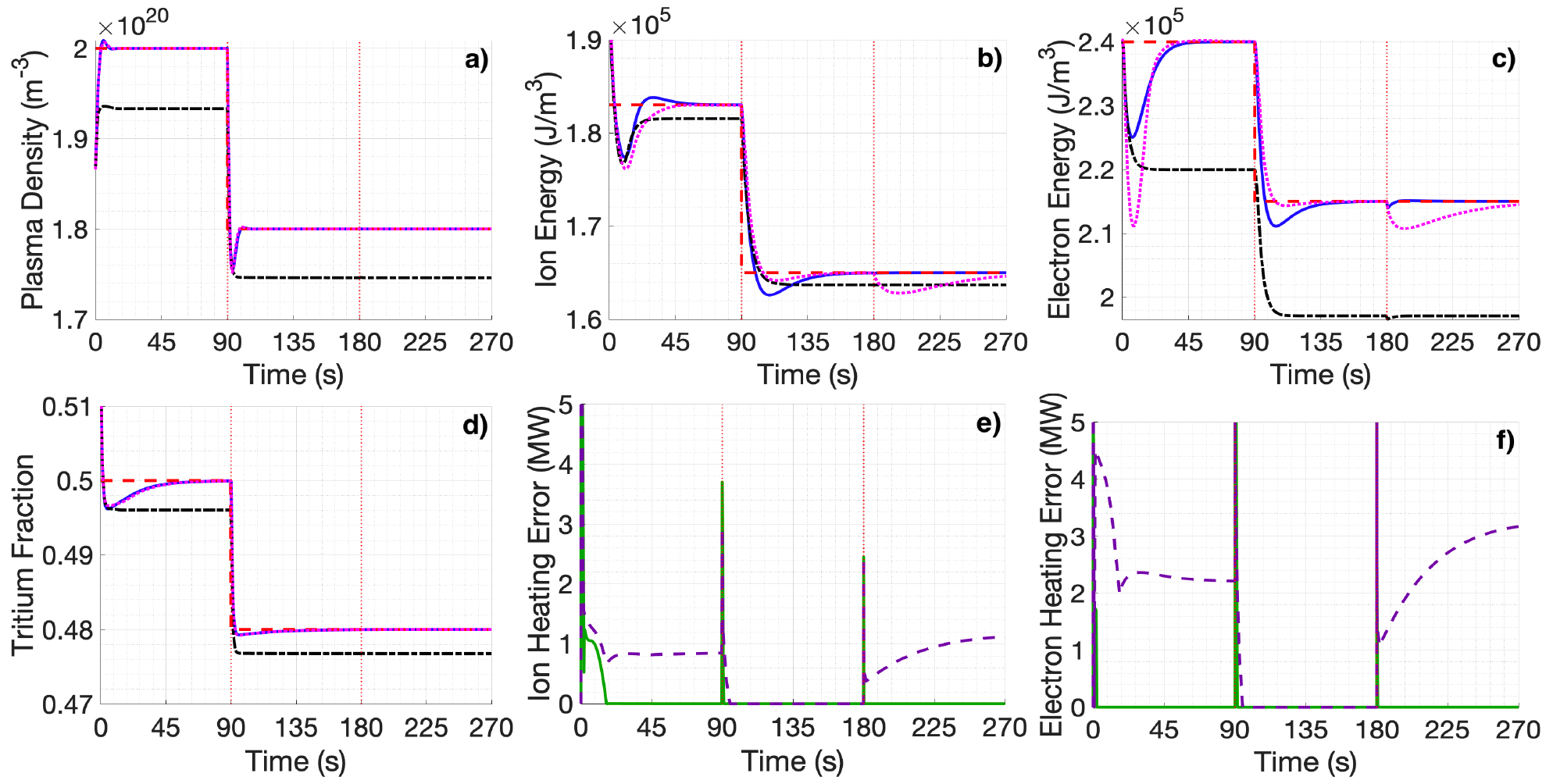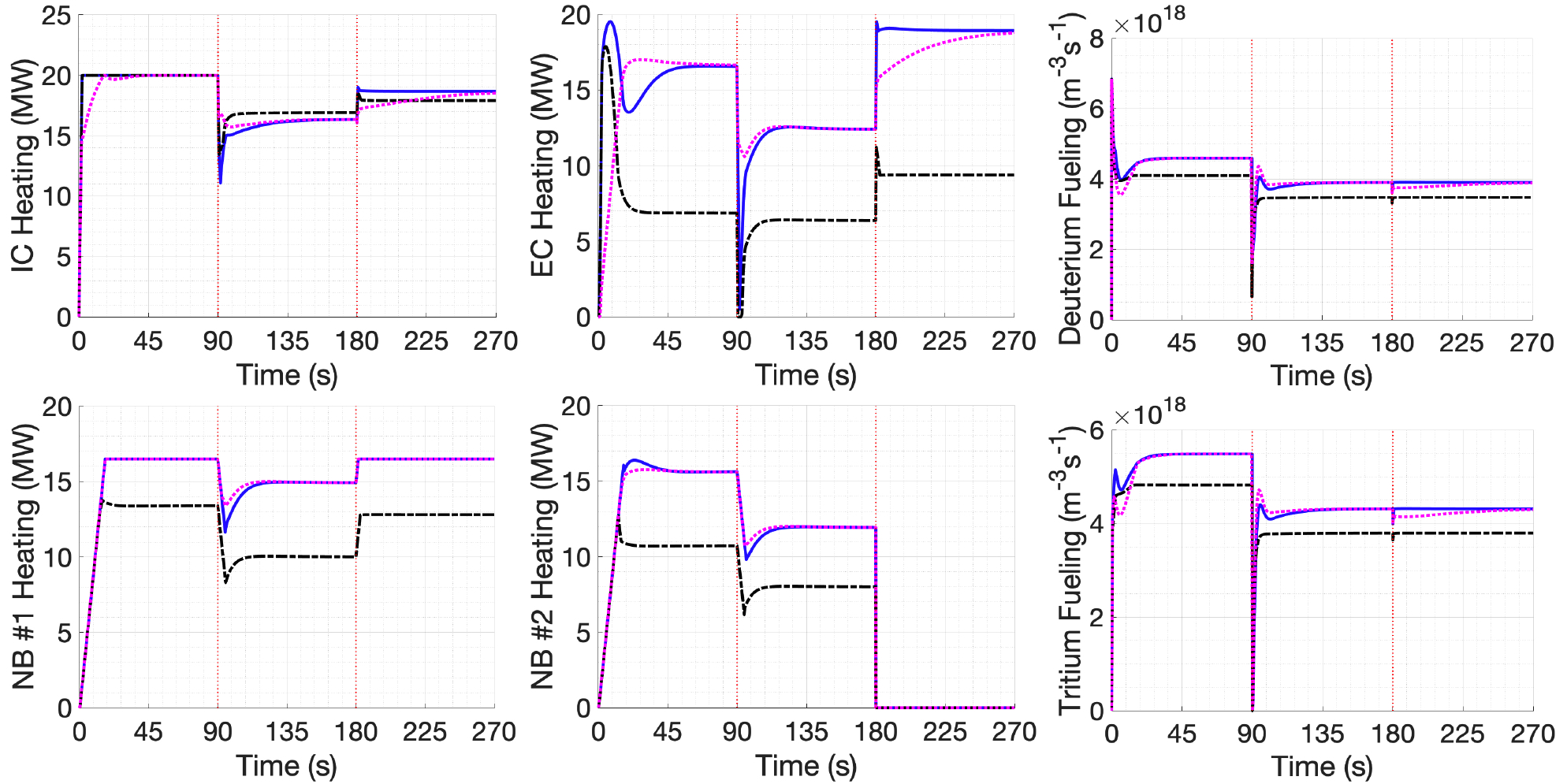Since 18 of December 2019 conferences.iaea.org uses Nucleus credentials. Visit our help pages for information on how to Register and Sign-in using Nucleus.

# 28th IAEA Fusion Energy Conference (FEC 2020)

10-15 May 2021
Virtual Event
Europe/Vienna timezone
The Conference will be held virtually from 10-15 May 2021

## Nonlinear Burn Control of ITER’s Two-temperature Plasmas Using Optimal and Adaptive Allocation of Actuators with Uncertain Dynamics

11 May 2021, 14:00
4h 45m
Virtual Event

#### Virtual Event

Regular Poster Magnetic Fusion Theory and Modelling

### Speaker

Vincent Graber (Lehigh University)

### Description

Successful operation of ITER will require robust regulation of the plasma temperature and density despite the plasma's nonlinear dynamics and various uncertainties. In this work, a burn-control algorithm was designed to determine control efforts that will drive the plasma to desired targets. In order to effectively achieve the commanded control efforts, control allocation modules were developed to optimally manage ITER's heating and fueling actuators. Using Lyapunov techniques, the nonlinear controller was synthesized from a zero-dimensional two-temperature model. For burning plasmas in ITER, the accuracy of the widely used scaling laws for the energy confinement time is unsure because they are based on experiments without alpha particle heating. Therefore, the proposed controller was designed with an adaptive estimation scheme to handle uncertainties in the plasma confinement and other complex phenomena (i.e. the fuel recycling and impurity sputtering that results from plasma-wall interactions). The plasma density is regulated by two control laws that request injection rates of deuterium and tritium. In ITER-like burning plasmas, the majority of the alpha particle heating will be deposited into the plasma electrons (ions receive less than $25\%$ of it). Consequently, the electron and ion temperatures will be uncoupled. The proposed controller independently regulates the ion and electron temperatures with separate control laws for the external ion and electron heating. Because the radiation losses depend on the electron temperature and the fusion reactivity depends on the ion temperature, independent temperature regulation permits plasma scenarios with improved performance.

ITER will have access to an ion cyclotron (IC) heating system ($20$ MW), an electron cyclotron (EC) heating system ($20$ MW) and two neutral beam (NB) injectors ($16.5$ MW each). With two commanded heating efforts (for the ions and electrons) and four heating actuators, an optimal control allocation (CA) module is necessary to map the commanded heating efforts to the heating actuators. The map can be written as $v=Bu$ where the vector $v$ contains the controller's commanded efforts, the vector $u$ contains the actuator allocation (power supplied by each actuator), and the control effectiveness matrix $B$ includes efficiency factors ($0-100\%$) for each actuator and the fractions of neutral beam heating that is delivered to the plasma ions and electrons. The allocator was designed with modularity such that it can be readily swapped out for another allocator. At each simulation time step, the proposed CA module solves an optimization problem that considers the saturation and rate limits of the heating actuators. The optimization problem was formulated as a strictly convex quadratic program (QP) that always permits a unique optimal solution. Since ITER will have only two fueling pellet injectors to satisfy the proposed controller's deuterium and tritium injection rate commands, CA is not required for density control.

For comparison to the QP CA module, a simpler pseudo-inverse CA module is introduced: $u=B^+ v$. To demonstrate performance of the adaptive control and CA algorithms, three simulations with different control schemes were generated. To assess the adaptive burn controller, an adaptive controller with QP CA is compared to a non-adaptive controller with QP CA. To assess the QP CA module, the adaptive controller with QP CA is also compared to an adaptive controller with pseudo-inverse CA. Each simulation uses the same plasma parameters, initial conditions, initial estimates of uncertain parameters, desired targets and actuator efficiencies. At $90$s, the desired target was changed. At $180$s, an actuator fault scenario was simulated by setting the actuator efficiency of the second neutral beam injector to zero. Fig. $1$ (a, b, c, d) shows how well the three control schemes can track the desired targets for plasma density, ion energy ($E_i$), electron energy ($E_e$), and tritium fraction (density of tritium over density of both deuterium and tritium) using the actuation shown in Fig. $2$. The adaptive controller with QP CA robustly stabilizes the equilibria even when the target changes and the second neutral beam injector shuts down. The non-adaptive controller with QP CA fails to track the target due to the modeled uncertainties. Unlike the adaptive controller with QP CA, the adaptive controller with pseudo-inverse CA cannot hold the plasma at the desired energy targets when the actuator fault occurs. By plotting the allocation errors in Fig. $1$ (e, f), the QP and pseudo-inverse CA modules can be better compared. The allocation error is the absolute value of the difference between the controller's commanded efforts and the control efforts produced from the allocated actuators. Allocation errors occur because either the commanded efforts are unattainable due to actuator constraints or the CA module fails to find the solution of $v=Bu$. The QP CA module can find a solution with zero allocation error throughout the simulation. In both the first-third and last-third of the simulation, the pseudo-inverse CA module fails to generate the commanded efforts by a few megawatts. The pseudo-inverse CA module's failures generate steady-state errors in the plasma energies (at $270$s $\Delta E_i=-1.12\times10^{5} \text{J}$ and $\Delta E_e=-1.42\times10^{5} \text{J}$).

An adaptive CA module with uncertainty in the allocation mapping ($B$) was also designed for the proposed adaptive burn controller. Unlike the previous QP CA module, this CA module considers the controller's commanded fueling efforts so that uncertainties in the tritium fraction of the fueling pellets can be handled. Furthermore, the actuator efficiency factors and the neutral beam heating fractions for the ions and electrons were considered uncertain. This adaptive CA module takes into account actuator dynamics, which also contain uncertainties, such as actuation lag times resulting from the thermalization delay of injected neutral beam particles, the ablation time for fueling pellets, ect. A Lyapunov function was used to formulate a dynamic update-law for $u$ (more computationally efficient than solving an optimization problem online).

This work has been supported in part by the U.S. Department of Energy under DE-SC0010661.Affiliation Lehigh University United States

### Primary authors

Vincent Graber (Lehigh University) Eugenio Schuster (Lehigh University)